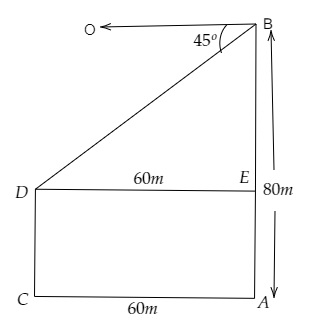# The horizontal distance between two trees of different heights is $60 \mathrm{~m}$. The angle of depression of the top of the first tree when seen from the top of the second tree is $45^{\circ}$. If the height of the second tree is $80 \mathrm{~m}$, find the height of the first tree.

Given:

The horizontal distance between two trees of different heights is $60 \mathrm{~m}$.

The angle of depression of the top of the first tree when seen from the top of the second tree is $45^{\circ}$.

The height of the second tree is $80 \mathrm{~m}$

To do:

We have to find the height of the first tree.

Solution:Let $AB$ and $CD$ be the two trees, the height of the second tree $AB=80\ m$.

Let the height of first pole $CD=h\ m$

Distance between the two poles, $AC = 60\ m$

From the figure,

$AC=DE= 60\ m$ and $AE=CD=h$ and $BE = 80-h\ m$

In $\vartriangle DBE$,

$tan45^{o}=\frac{BE}{DE}$

$\Rightarrow 1 =\frac{80-h}{60}$

$\Rightarrow 60=80-h$

$\Rightarrow h=80-60$

$\Rightarrow h=20\ m$

Therefore, the height of the first tree is $20\ m$.